## Audio Stream Service Class Diagram

6
0
0
publish time: 2021-05-09WSlEkWla

Here is a class diagram about the audio stream service. A class represents a set of objects that share common characteristics, namely behavior, and structure. A rectangular symbol split shows a class into three sections: class name, attributes, and operations. Learn more details from this UML class diagram, and try to make yours with ease.

See More Related TemplatesThis is an example of a UML diagram about adding events to the calendar.
UML Diagram - Add event to calendar
23
1
0easy diagrams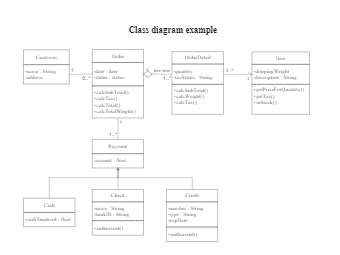This is an example of a uml class diagram.
Class diagram example
199
6
4easy diagrams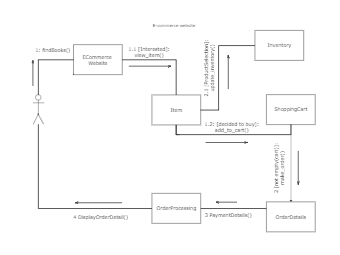This is an example of a UML collaboration diagram.
ecommerce website - UML diagram
24
2
0easy diagrams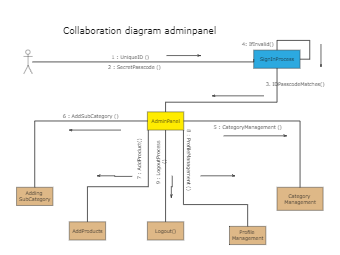11
1
0easy diagrams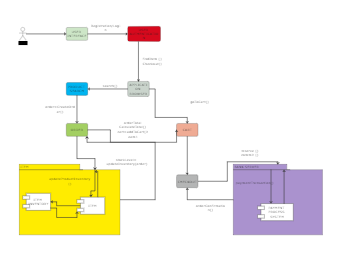This is an example of a UML communication diagram.
Communication diagram example
11
1
1easy diagrams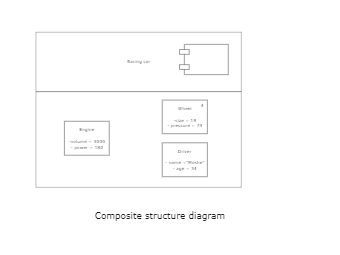This is an example of a composite structure diagram.
Composite structure diagram
3
0
0easy diagrams